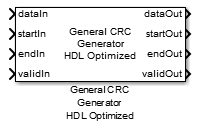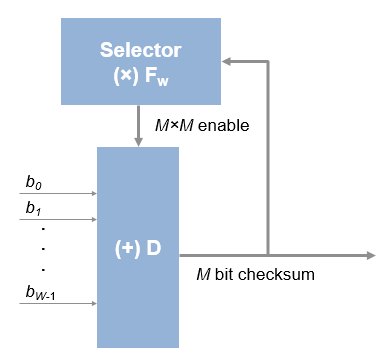General CRC Generator HDL Optimized

Generate CRC code bits and append them to input data

• Library:
• Communications Toolbox HDL Support / Error Detection and Correction / CRC

Communications Toolbox / Error Detection and Correction / CRC

•Description

The General CRC Generator HDL Optimized block, which is similar to the General CRC Generator block, generates a cyclic redundancy check (CRC) checksum and appends it to the input message. The General CRC Generator HDL Optimized block processing is optimized for HDL code generation. Instead of processing an entire frame at once, the block accepts and returns a data sample stream with accompanying control signals. The control signals indicate the validity of the samples and the boundaries of the frame. To achieve higher throughput, the block accepts vector data up to the CRC length and implements a parallel architecture.

Ports

Input

expand all

Input data, specified as one of these options.

• Scalar – Specify an integer representing several bits. For this case, the block supports an unsigned integer (uint8, uint16, or uint32) or fixdt(0,N,0) data type.

• Vector – Specify a vector of binary values. For this case, the block supports a double or Boolean data type.

The data width must be less than or equal to the CRC length, and the CRC length must be divisible by the data width. For CRC-CCITT/CRC-16, the valid data widths are 16, 8, 4, 2, and 1.

Example: The uint8 vector input [0 0 0 1 0 0 1 1] is equivalent to 19.

Data Types: double | uint8 | uint16 | uint32 | fixed point | Boolean

Start of input frame indicator, specified as a Boolean scalar.

Data Types: Boolean

End of input frame indicator, specified as a Boolean scalar.

Data Types: Boolean

Valid input data indicator, specified as a Boolean scalar.

This is a control signal that indicates if the data on the dataIn port is valid.

Data Types: Boolean

Output

expand all

Output data with appended checksum, returned as a scalar or vector. The output data type and size are the same as the input data.

Data Types: double | uint8 | uint16 | uint32 | Boolean | fixed point

Start of output frame indicator, returned as a Boolean scalar.

Data Types: Boolean

End of output frame indicator, returned as a Boolean scalar.

Data Types: Boolean

Valid output data indicator, returned as a Boolean scalar.

This port is a control signal that indicates if the data on the dataOut port is valid.

Data Types: Boolean

Parameters

expand all

Specify the generator polynomial as a binary vector with coefficients in descending order of powers. The vector length is equal to the degree of the polynomial plus 1.

Specify initial conditions of the internal shift register as a binary, double-precision, or single-precision scalar or vector. For vector inputs, the length of the initial state must be equal to the degree of the generator polynomial.

Specify the direct or indirect method for calculating the checksum.

• Select this parameter for the block to use the direct algorithm for CRC checksum calculations.

• Clear this parameter for the block to use the nondirect algorithm for CRC checksum calculations.

Specify the input byte order.

• Select this parameter for the block to flip each input byte before it enters the shift register.

• Clear this parameter for the block to pass the message data to the shift register unchanged.

The input data width must be a multiple of 8.

Specify the checksum byte order.

• Select this parameter for the block to flip each checksum byte before passing it to the final XOR stage.

• Clear this parameter for the block to pass the checksum byte to the final XOR stage unchanged.

The input data width must be a multiple of 8.

Specify the checksum as a binary, double-precision, or single-precision data type scalar or vector. The block performs XOR operation on the CRC checksum with this value before appending it to the input data.

If you specify a vector input, the vector length must be equal to the degree of the generator polynomial.

Algorithms

expand all

When you use a vector or integer input, the block implements a parallel CRC algorithm .

To provide high throughput for modern communications systems, the block implements the CRC algorithm with a parallel architecture. This architecture recursively calculates M bits of a CRC checksum for each W input bits. At the end of the frame, the final checksum result is appended to the message. For a polynomial length of M, the recursive checksum calculation for W bits in parallel is

${X}^{\text{'}}={F}_{W}\left(×\right)X\left(+\right)D.$

FW is an M-by-M matrix that selects elements of the current state for the polynomial calculation with the new input bits. D is an M-element vector that provides the new input bits, ordered in relation to the generator polynomial and padded with zeros. The block implements the (×) with logical AND and (+) with logical XOR.Campobello, G., G. Patane, and M. Russo. “Parallel Crc Realization.” IEEE Transactions on Computers 52, no. 10 (October 2003): 1312–19. https://doi.org/10.1109/TC.2003.1234528.